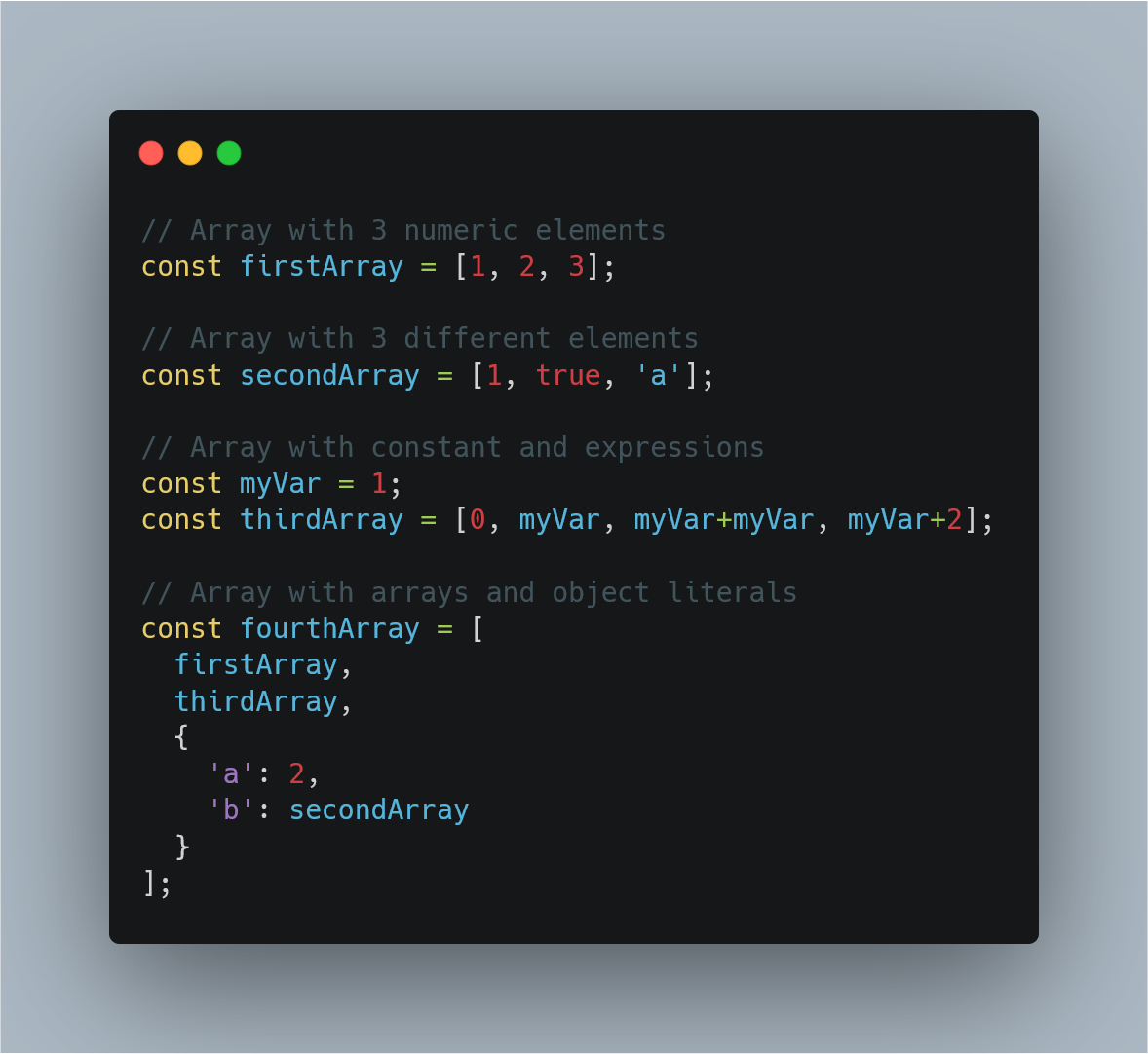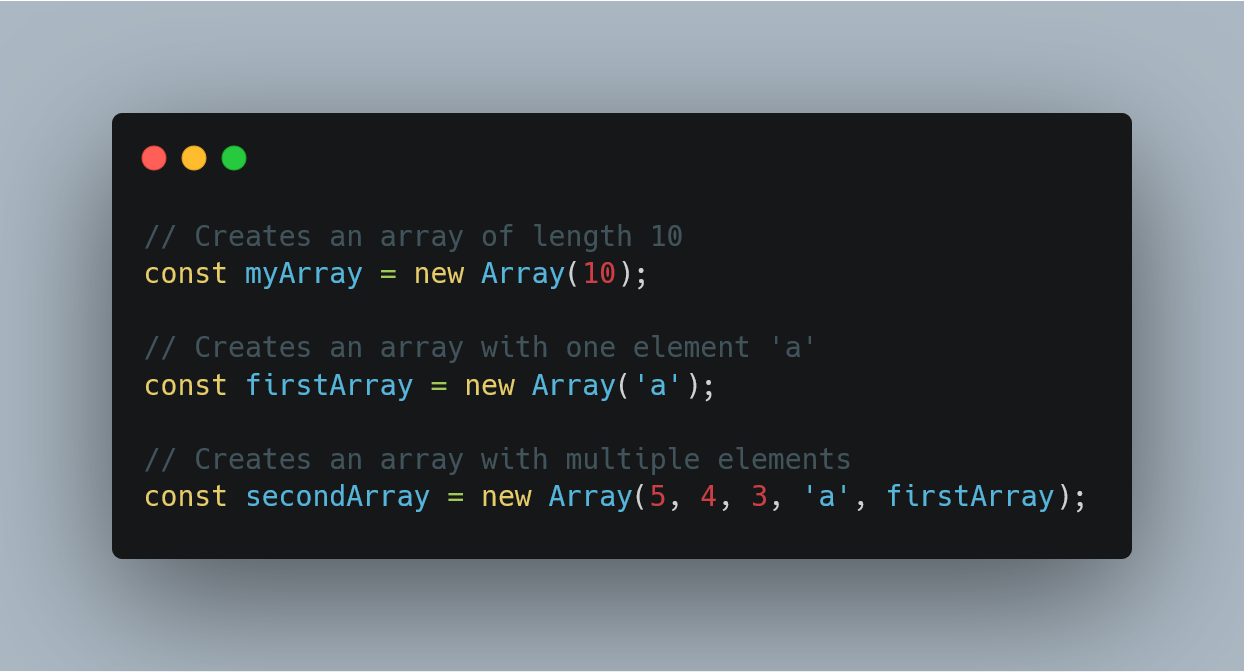1612409376

# Randomly Extracting a Value from an Array in Javascript

In one of the comments recently there was a question about randomly extracting values from an array. As I looked through my tutorials, I discovered I haven’t done a tutorial on random numbers. But to make it more than that, I want to do a tutorial on a generator function, randomly retrieving values from an array until they are all gone.

#js #javascript

## Buddha Community1624388400

## JavaScript Arrays Tutorial. DO NOT MISS!!!

Learn JavaScript Arrays

📺 The video in this post was made by Programming with Mosh
The origin of the article: https://www.youtube.com/watch?v=oigfaZ5ApsM&list=PLTjRvDozrdlxEIuOBZkMAK5uiqp8rHUax&index=4
🔥 If you’re a beginner. I believe the article below will be useful to you ☞ What You Should Know Before Investing in Cryptocurrency - For Beginner
⭐ ⭐ ⭐The project is of interest to the community. Join to Get free ‘GEEK coin’ (GEEKCASH coin)!
Thanks for visiting and watching! Please don’t forget to leave a like, comment and share!

#arrays #javascript #javascript arrays #javascript arrays tutorial1602154740

## Fill and Filter in Array in JavaScript

By the word Array methods, I mean the inbuilt array functions, which might be helpful for us in so many ways. So why not just explore and make use of them, to boost our productivity.

Let’s see them together one by one with some amazing examples.

### Array.fill():

The `_fill()_` method changes all elements in an array to a static value, from a start index (default `_0_`) to an end index (default `_array.length_`). It returns the modified array.

In simple words, it’s gonna fill the elements of the array with whatever sets of params, you pass in it. Mostly we pass three params, each param stands with some meaning. The first param value: what value you want to fill, second value: start range of index(inclusive), and third value: end range of index(exclusive). Imagine you are going to apply this method on some date, so that how its gonna look like eg: array.fill(‘Some date’, start date, end date).

``````NOTE: Start range is inclusive and end range is exclusive.
``````

Let’s understand this in the below example-

``````//declare array
var testArray = [2,4,6,8,10,12,14];

console.log(testArray.fill("A"));
``````

When you run this code, you gonna see all the elements of `testArray` will be replaced by `'A'` like `[“A”,"A","A","A","A","A","A"]`.

#javascript-tips #array-methods #javascript-development #javascript #arrays1597470780

## Introduction

Arrays are a structure common to all programming languages so knowing what they are and having a firm grasp on what you’re able to accomplish with Arrays will take you a long way in your journey as a software developer. The code examples I share in this post will be in JavaScript but the concepts are common among all languages. What you learn here can easily be translated to any other language you work with.

In this post I’ll be covering how to perform the create, read update and delete operations using arrays, some common functions that come with the Array prototype and also how to implement them.

## What is an Array

Before we jump into the juicy bits of Arrays, lets quickly gloss over what they are. Arrays

• are a fundamental data type in JavaScript
• are an ordered collection of values called **elements **that are stored at and accessed via an index
• are untyped, meaning that the elements of an array could be of different types. This allows us to create complex arrays such as an array of objects or even an array of arrays (multidimensional arrays)
• can have elements that are constants or expressions
• have a property called length that tells you the number of elements in the array
• inherit properties from `Array.prototype` that includes a wide variety useful functions that can be called from arrays or `array-like` objects

## CRUD operations using Arrays

If you’re not familiar with the term CRUD it stands for Create, Read, Update and Delete. In this section we’ll go through each one of these operations and cover different ways you can perform each one.

### Creating Arrays

There are several ways you can create an Array but the most common ways are by using

• the Array literal syntax
• the Array constructor i.e. `new Array()`

Lets take a look at each one with examples

### Array literal

The array literal is the most common way of creating an array. It uses the square brackets as a notion of a container followed by comma separated values inside the square brackets. The following examples show how to use the array literal syntax and how arrays are untyped i.e. can contain elements of different types.Examples of untyped arrays in JavaScript created with the array literal syntax.

### Array constructor

Another way to create an array is through the Array constructor.

``````const myArray = new Array();
``````

Using the Array constructor, as shown above, is the same as creating an array with the array literal syntax. i.e.

``````// The following two lines behave exactly the same way i.e. both create an empty arrays

const myArray = new Array();
const myOtherArray = [];
``````

The array constructor, however, is able to receive arguments that allow it to behave in different ways depending on the number and type of arguments passed to it.

• You can pass a single numeric argument which creates an array of the specified length. This option is mostly used when you know how many elements you’ll be placing in the array
``````const myArray = new Array(5);
``````

Note: If you want to define the array with a specified size, as shown above, the argument passed must be a numeric value. Any other type would be considered as the first element that’ll be placed in the array.

• Or you can pass two or more arguments or a non-numeric argument to place the values inside the array. This works the same way as shown in the array literal examples.Examples of arrays created by using the Array constructor in JavaScript

As stated earlier, these two ways are the most common ways of creating arrays that you’ll see and use 99% of the time. There are a few other ways but we won’t dive deep into how they work. They are

• the spread operator `const someArray = […someOtherArray]`
• the static method `Array.of()`
• and the static method `Array.from()`

#javascript #web-development #javascript-tips #javascript-development #javascript-arrays #sql1600510680

## Definitive Guide to Understand Javascript Array reduce()

Javascript array reduce() is an inbuilt method that is used to apply a function to each element in the array to reduce the array to a single value. The reduce() function executes the provided function for each value of an array from left-to-right. The return value of a function is stored in an accumulator.

### Understanding Javascript array reduce()

JavaScript array reduce() is one of the pioneer function of functional programming. The reduce() method accepts two parameters, the total and the current value. If you want to add all the values of an array, then use the array reduce() function.

It is similar to both  Javascript map() and  Javascript filter() but, it differs in the callback arguments.

The callback now receives an accumulator (it accumulates all the return values. Its value is the accumulation of a previously returned accumulations), a current value, a current index, and finally, the whole array.

#javascript #javascript map #javascript filter #javascript array reduce1600410360

## Javascript Array From Example | Array.prototype.from()

Javascript array from() is an inbuilt function that creates a new, shallow-copied array instance from an array-like object or iterable object.

The Array .from() lets you develop Arrays from the array-like objects (objects with a length property and indexed items) or  iterable objects ( objects where you can get its items, such as Map and  Set).

The Array from() function was introduced in ECMAScript 2015.

### Javascript Array From Example

Array.from() method in Javascript is used to creates a new  array instance from a given array. If you pass a  string to the Array.from() function, then, in that case, every alphabet of the string is converted to an element of the new array instance. In the case of integer values, a new array instance simply takes the elements of the given Array.

The syntax of the Array.from() method is the following.

#### Syntax

``````Array.from(arrayLike[, mapFn[, thisArg]])
``````

#javascript #ecmascript #javascript array from #array.prototype.from